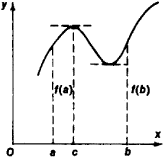Rolle's Theorem

(redirected from Rolle theorem)
Also found in: Dictionary.
Related to Rolle theorem: Mean value theorem

Rolle's theorem

[′rȯlz ‚thir·əm]
(mathematics)
If a function ƒ(x) is continuous on the closed interval [a, b ] and differentiable on the open interval (a, b) and if ƒ(a) = ƒ(b), then there exists x0, a <>x0<>b, such that ƒ′(x 0)=0.

Rolle’s Theorem

a theorem of mathematical analysis first stated by M. Rolle in 1690. According to this theorem, if the function f(x) is continuous on the closed interval [a, b], has a definite derivative within the interval, and takes on the equal values f(a) = f(b) at the ends of the interval, then the function’sFigure 1

derivative f’(x) vanishes at least once in the interval (a, b)—that is, there exists a c, a < c < b, such that f’(c) = 0. A corollary of the theorem provides that the derivative of a function has at least one zero between two successive zeros of the function. Geometrically, Rolle’s theorem is self-evident (see Figure 1). (See alsoDIFFERENTIAL CALCULUS.)

Site: Follow: Share:
Open / Close